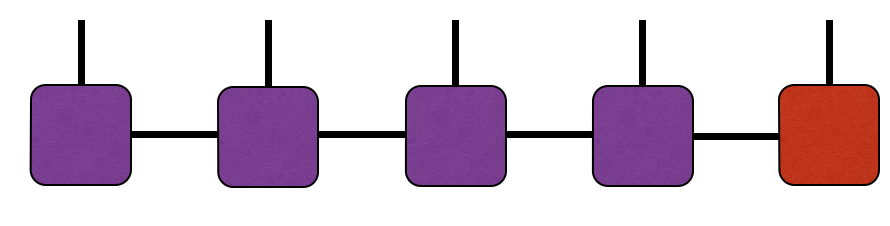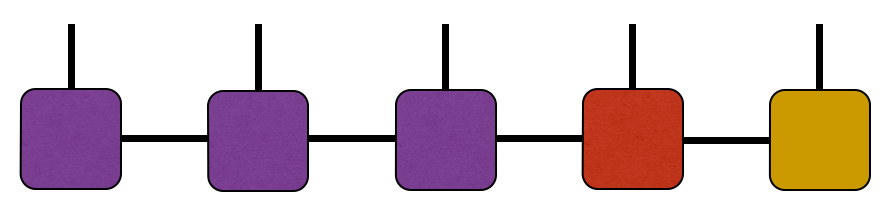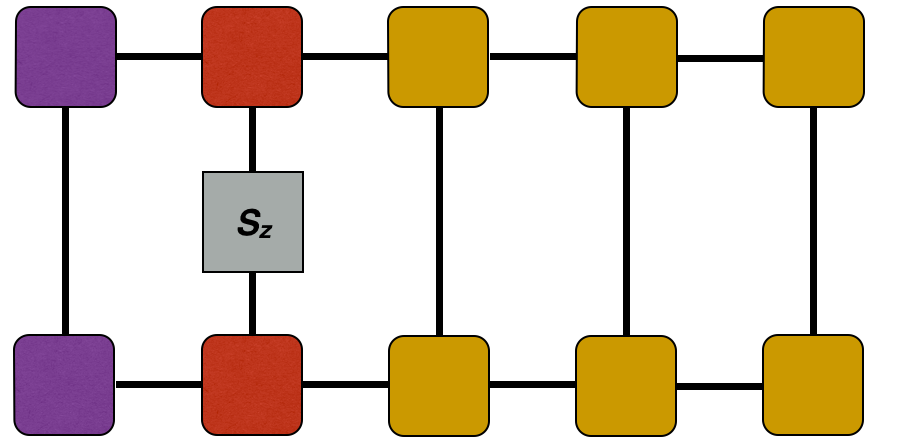## Learn to Use ITensor

main / tutorials / MPS C++v3 | C++v2 | Julia

Matrix Product States

Thomas E. Baker—August 17, 2015

The Hilbert space is large. Scanning through the entire space would be take longer than is reasonable. Writing down a good guess for the wavefunction can be a great way to get close enough to the right ground state. A very good guess for the ground state of a gapped system in one dimension is the matrix product state (MPS). DMRG and many tensor network methods rely on the MPS ansatz to represent ground states and low-lying excited states.

Each bulk tensor of an MPS has three indices, $A^{\sigma_i}_{a_i a_{i+1}}$ . The subscripts convey that indices connecting different bonds may vary in size. The size of a typical index $a_i$ is known as the bond dimension of the MPS, and corresponds to the number of states kept in a DMRG calculation. The index $\sigma_i$ is the physical index. This has two values for a spin $-1/2$ chain.

An MPS diagram for a five site system looks likeWe will discuss some characteristics of MPSs and introduce some concepts that are essential for working with MPS in ITensor.

## Using the ITensor MPS Class

To make an MPS, one typically uses the following code pattern:

SpinHalf sites(5);
auto psi = MPS(sites);//construct a random product state with indices
//based on the SiteSet used (here SpinHalf)


which automatically makes a random product-state MPS. Here we chose the Hilbert space to be spin 1/2 sites, but other types of sites are available (see the list of site sets on this page).

For example, to make a generic five-site MPS where each site has a local dimension (physical index dimension) of two:

SiteSet sites(5,2);
auto psi = MPS(sites);


For read-only access to a particular tensor of the MPS, use the psi.A(j) method. This method is called "A" because it is very common in the MPS literature to use the letter A to denote MPS tensors. For example, if we want to print the fourth tensor of the above MPS, we could do

Print(psi.A(4));


For read and write access to an MPS tensor, use the psi.Aref(j) method. This method is called "Aref" because it returns a reference to the j'th "A" tensor. Here is an example of using this method to multiply the third MPS tensor by a scalar:

psi.Aref(3) *= 0.1827;


Another way to modify the tensors of an MPS is to use the .setA method.

## Working with MPS Gauges

The gauge of an MPS can be chosen to make computing local properties very efficient. The gauge used in standard DMRG calculations chooses a certain site to be the orthogonality center. All sites to the left and right of the orthogonality center will be left and right orthogonal, respectively.

To set site 4 of the 5-site MPS above to be the orthogonality center, call

psi.position(4);


ITensor will compute a series of singular value decompositions (in this case, only one) until the orthogonality center reaches site 4. The MPS diagram now looks like:To illustrate one advantage of setting the MPS gauge, consider the diagram below where we have drawn purple squares to be left-normalized while yellow squares are right-normalized.First call psi.position(2) to center the gauge at site 2 as in the above diagram.

Then to compute the expectation value of the "Sz" operator on site 2, we can use the fact that in the diagram above all of the left-orthogonal and right-orthogonal tensors contract to the identity, so can be left out of the calculation (see an article on Correlation functions for more details). So the only code needed to obtain the expectation value is:

auto Mz2 = (dag(prime(psi.A(2),Site))*sites.op("Sz",2)*psi.A(2)).real();


where notice that we only accessed the tensor on site 2 and no other MPS tensors. We need not bother with the other sites since we know they contract to the identity.Back to TutorialsBack to Main